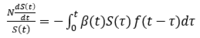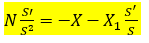# Biological Model

#### TOIDIS

##### New member
I am developing a biological model and stuck in this differential equation:

X'/y' - X/y = N ............ edited per OP​

Thanks.
where y and X are functions of x, while y' and X' are the respective derivatives regarding x.​
Can someone help me?
TOIDIS

Last edited by a moderator:

#### Subhotosh Khan

##### Super Moderator
Staff member
I am developing a biological model and stuck in this differential equation:

X'/y' - X/y = N ............ edited per OP​

Thanks.
where y and X are functions of x, while y' and X' are the respective derivatives regarding x.​
Can someone help me?
TOIDIS
Please show us what you have tried and exactly where you are stuck.

Please follow the rules of posting in this forum, as enunciated at:

Please share your work/thoughts about this problem.

#### TOIDIS

##### New member
Well, the original equation is a little bit more complicated, and I have wandered a lot facing desert mirages and jungle beasts. And finally I thought I had settled in an oasis in that equation. But there was no water over there. So, the initial problem is this equationIn this equation beta and f are given. The task is to find S(t)
I made some variable change and I have found an equivalent equation, which is:In this equation S and S' were replaced for y and y' in my first post

I tried to make 1/s=w and arrived to X'/y' - X/y = N

#### Attachments

• 1.3 KB Views: 1